Courses

# Chapter Notes - Practical Geometry Class 6 Notes | EduRev

## Class 6: Chapter Notes - Practical Geometry Class 6 Notes | EduRev

The document Chapter Notes - Practical Geometry Class 6 Notes | EduRev is a part of the Class 6 Course Mathematics (Maths) Class 6.
All you need of Class 6 at this link: Class 6

14. Practical Geometry

Basic Constructions: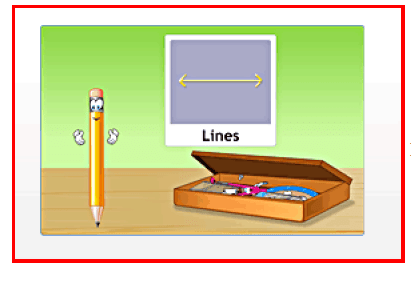Ruler, Compass, Divider, Set squares, Protractor.

The tools in our geometry box are:
• Ruler
• Compass
• Divider
• Set squares
• Protractor

The description of each tool and its uses are given below:

Ruler:
A ruler is a flat and straight-edged strip, whose one side is graduated into centimetres and the other into inches. A ruler is commonly called a scale. It is the most essential tool in geometry. It is used in all constructions.

The basic uses of a ruler are:

• Measuring lengths of line segments.
• Drawing line segments.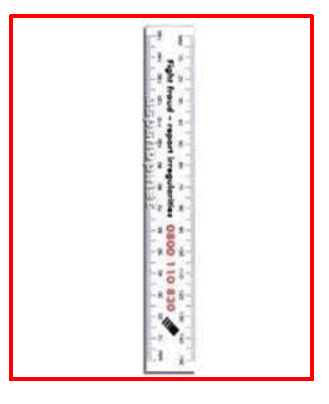Page 58

Compass:

A compass has two ends. One end holds a pointer, while the other end holds a pencil. It is also called a pair of compasses.
The basic uses of a compass are:

• Marking off equal lengths.
• Drawing arcs.
• Drawing circles.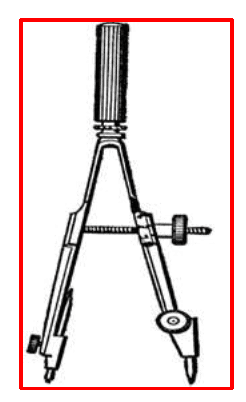Divider:

A divider is a tool similar in shape to a compass. It has a pair of pointer ends.

Page 59

The basic uses of a divider are:

• Comparing lengths of line segments.
• Helping avoid positioning errors.
• Taking accurate measurements.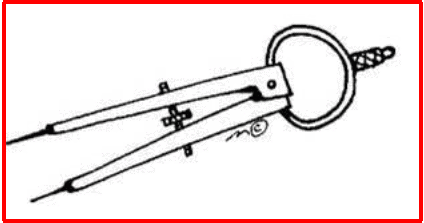Set squares:

The two triangular tools in the geometry box are called set squares. One of the set square is an isosceles triangle with two angles measuring 45° each. The other set square is a scalene triangle with two angles measuring 30 °and 60° each. The two perpendicular sides of either set square are graduated into centimetres.

The basic uses of set squares are:

• Drawing perpendicular lines.
• Drawing parallel lines.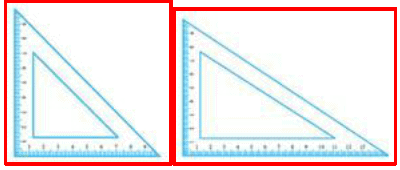Protractor:

A semi-circular tool with degrees marked is called a protractor. The centre of the semicircle is called the midpoint of the protractor. This point helps as a reference point for the protractor. The horizontal line is called the base line or the straight edge of the protractor.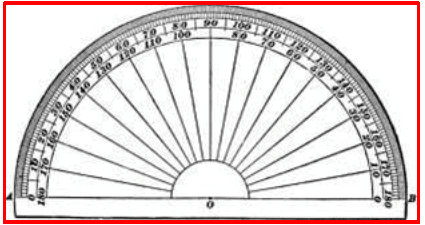Page 60

The basic uses of a protractor are:

• Measuring angles.
• Drawing angles.

The important points to be remembered while using the tools for construction are:

• Draw smooth and thin lines.
• Mark points lightly.
• Maintain tools or instruments with sharp pointers and fine edges.
• Keep two pencils in the box. One is for drawing lines and marking points. The other is for using in the compass.

Construction of Lines:Steps to construct a line segment of length 5 cm

Steps to construct a line segment of length 5 cm:

 Draw line 1.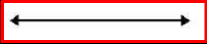Mark a point on line l and name it P.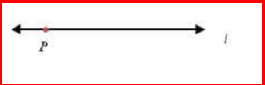Open the compass to measure the length of the line segment by placing the pointer on the 0 mark of the ruler and the pencil point on the 5 cm mark.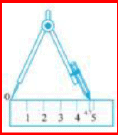Place tlie pointer of the compass on point P.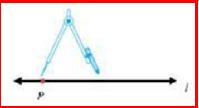Swing an arc oil tlie line to cut it at Q.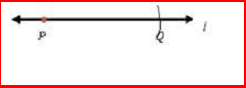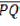is the required line segment of length 5 cm.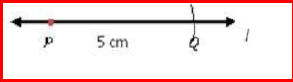Two lines are said to be perpendicular when they intersect each other at an angle of 90o.

The perpendicular bisector is a perpendicular line that bisects another line into two equal parts.

Constructing of Angles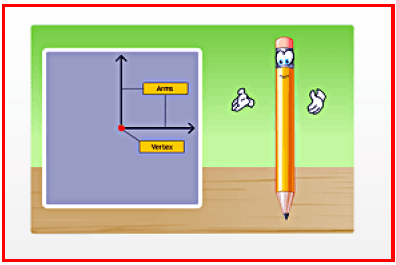An exact copy of a line segment can be constructed using a ruler and a compass.

An exact copy of a line segment can be constructed using a ruler and a compass.

To construct a copy of an angle:
• Draw a line AB.
• Mark any point O on AB.

Page 62

• Place the compass pointer at vertex X of the given figure and draw an arc with a convenient radius, cutting rays XY and XZ at points E and F, respectively.
• Without changing the compass settings, draw an arc on line AB from point O. It cuts line AB at P.
• Set the compass to length EF.
• Without changing the compass settings, draw an arc from P cutting the previous arc at point Q.
• Join points O and Q.
• Hence, ∠POQ is the required copy of ∠YXZ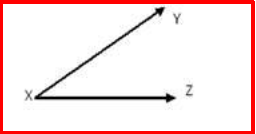To construct the bisector of an angle:

Let the given angle be LMN.
Place the compass pointer at vertex M of the given angle.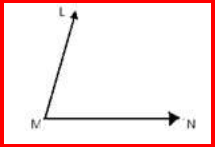Draw an arc cutting rays ML and MN at U and V, respectively.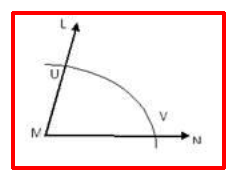Draw an arc with V as the centre and a radius more than half the length of UV in the interior of ∠LMN.

Page 63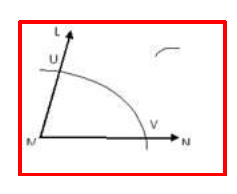Draw another arc with U as the centre and the same radius intersecting the previous arc.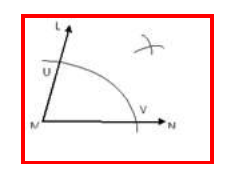Name the point of intersection of the arcs as X.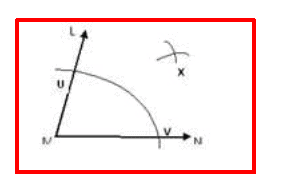Join points M and X.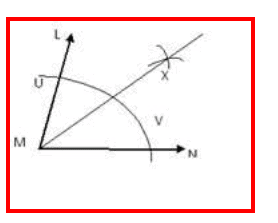Thus, the ray MX is the required bisector of ∠LMN

In a similar way, we can construct:
• A 60° angle without using the protractor.
• A 120° angle without using the protractor.
• A 90° angle without using the protractor.

The document Chapter Notes - Practical Geometry Class 6 Notes | EduRev is a part of the Class 6 Course Mathematics (Maths) Class 6.
All you need of Class 6 at this link: Class 6Use Code STAYHOME200 and get INR 200 additional OFF Use Coupon Code
All Tests, Videos & Notes of Class 6: Class 6

### Top Courses for Class 6## Mathematics (Maths) Class 6

185 videos|229 docs|43 tests

### Top Courses for Class 6Track your progress, build streaks, highlight & save important lessons and more!

,

,

,

,

,

,

,

,

,

,

,

,

,

,

,

,

,

,

,

,

,

;